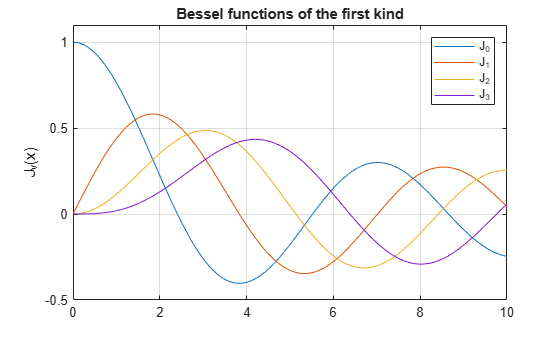# besselj

Bessel function of the first kind for symbolic expressions

## Syntax

``besselj(nu,z)``

## Description

example

````besselj(nu,z)` returns the Bessel function of the first kind, Jν(z).```

## Examples

### Find Bessel Function of First Kind

Compute the Bessel functions of the first kind for these numbers. Because these numbers are floating point, you get floating-point results.

`[besselj(0,5) besselj(-1,2) besselj(1/3,7/4) besselj(1,3/2+2*i)]`
```ans = -0.1776 + 0.0000i -0.5767 + 0.0000i 0.5496 + 0.0000i 1.6113 + 0.3982i```

Compute the Bessel functions of the first kind for the numbers converted to symbolic form. For most symbolic (exact) numbers, `besselj` returns unresolved symbolic calls.

```[besselj(sym(0),5) besselj(sym(-1),2)... besselj(1/3,sym(7/4)) besselj(sym(1),3/2+2*i)]```
```ans = [ besselj(0, 5), -besselj(1, 2), besselj(1/3, 7/4), besselj(1, 3/2 + 2i)]```

For symbolic variables and expressions, `besselj` also returns unresolved symbolic calls.

```syms x y [besselj(x,y) besselj(1,x^2) besselj(2,x-y) besselj(x^2,x*y)]```
```ans = [ besselj(x, y), besselj(1, x^2), besselj(2, x - y), besselj(x^2, x*y)]```

### Solve Bessel Differential Equation for Bessel Functions

Solve this second-order differential equation. The solutions are the Bessel functions of the first and the second kind.

```syms nu w(z) ode = z^2*diff(w,2) + z*diff(w) +(z^2-nu^2)*w == 0; dsolve(ode)```
```ans = C2*besselj(nu, z) + C3*bessely(nu, z)```

Verify that the Bessel function of the first kind is a valid solution of the Bessel differential equation.

```cond = subs(ode,w,besselj(nu,z)); isAlways(cond)```
```ans = logical 1```

### Special Values of Bessel Function of First Kind

Show that if the first parameter is an odd integer multiplied by 1/2, `besselj` rewrites the Bessel functions in terms of elementary functions.

```syms x besselj(1/2,x)```
```ans = (2^(1/2)*sin(x))/(x^(1/2)*pi^(1/2))```
`besselj(-1/2,x)`
```ans = (2^(1/2)*cos(x))/(x^(1/2)*pi^(1/2))```
`besselj(-3/2,x)`
```ans = -(2^(1/2)*(sin(x) + cos(x)/x))/(x^(1/2)*pi^(1/2))```
`besselj(5/2,x)`
```ans = -(2^(1/2)*((3*cos(x))/x - sin(x)*(3/x^2 - 1)))/(x^(1/2)*pi^(1/2))```

### Differentiate Bessel Function of First Kind

Differentiate expressions involving the Bessel functions of the first kind.

```syms x y diff(besselj(1,x))```
```ans = besselj(0, x) - besselj(1, x)/x ```
`diff(diff(besselj(0,x^2+x*y-y^2), x), y)`
```ans = - besselj(1, x^2 + x*y - y^2) -... (2*x + y)*(besselj(0, x^2 + x*y - y^2)*(x - 2*y) -... (besselj(1, x^2 + x*y - y^2)*(x - 2*y))/(x^2 + x*y - y^2))```

### Find Bessel Function for Matrix Input

Call `besselj` for the matrix `A` and the value 1/2. `besselj` acts element-wise to return matrix of Bessel functions.

```syms x A = [-1, pi; x, 0]; besselj(1/2, A)```
```ans = [ (2^(1/2)*sin(1)*1i)/pi^(1/2), 0] [ (2^(1/2)*sin(x))/(x^(1/2)*pi^(1/2)), 0]```

### Plot Bessel Functions of First Kind

Plot the Bessel functions of the first kind for $0,1,2,3$.

```syms x y fplot(besselj(0:3, x)) axis([0 10 -0.5 1.1]) grid on ylabel('J_v(x)') legend('J_0','J_1','J_2','J_3', 'Location','Best') title('Bessel functions of the first kind')```## Input Arguments

collapse all

Input, specified as a number, vector, matrix, or array, or a symbolic number, variable, array, function, or expression.

If `nu` is a vector or matrix, `besselj` returns the modified Bessel function of the first kind for each element of `nu`.

Input, specified as a number, vector, matrix, or array, or a symbolic number, variable, array, function, or expression.

If `nu` is a vector or matrix, `besselj` returns the modified Bessel function of the first kind for each element of `nu`.

collapse all

### Bessel Functions of the First Kind

The Bessel functions are solutions of the Bessel differential equation.

`${z}^{2}\frac{{d}^{2}w}{d{z}^{2}}+z\frac{dw}{dz}+\left({z}^{2}-{\nu }^{2}\right)w=0$`

These solutions are the Bessel functions of the first kind, Jν(z), and the Bessel functions of the second kind, Yν(z).

`$w\left(z\right)={C}_{1}{J}_{\nu }\left(z\right)+{C}_{2}{Y}_{\nu }\left(z\right)$`

This formula is the integral representation of the Bessel functions of the first kind.

`${J}_{\nu }\left(z\right)=\frac{{\left(z/2\right)}^{\nu }}{\sqrt{\pi }\Gamma \left(\nu +1/2\right)}\underset{0}{\overset{\pi }{\int }}\mathrm{cos}\left(z\mathrm{cos}\left(t\right)\right)\mathrm{sin}{\left(t\right)}^{2\nu }dt$`

## Tips

• Calling `besselj` for a number that is not a symbolic object invokes the MATLAB® `besselj` function.

• At least one input argument must be a scalar or both arguments must be vectors or matrices of the same size. If one input argument is a scalar and the other one is a vector or a matrix, `besselj(nu,z)` expands the scalar into a vector or matrix of the same size as the other argument with all elements equal to that scalar.

 Olver, F. W. J. “Bessel Functions of Integer Order.” Handbook of Mathematical Functions with Formulas, Graphs, and Mathematical Tables. (M. Abramowitz and I. A. Stegun, eds.). New York: Dover, 1972.

 Antosiewicz, H. A. “Bessel Functions of Fractional Order.” Handbook of Mathematical Functions with Formulas, Graphs, and Mathematical Tables. (M. Abramowitz and I. A. Stegun, eds.). New York: Dover, 1972.

## Support

#### Mathematical Modeling with Symbolic Math Toolbox

Get examples and videos